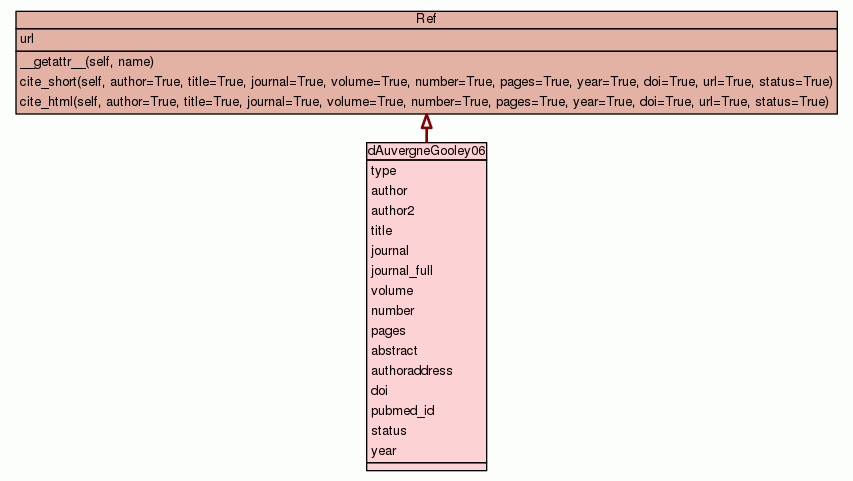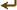[frames] | no frames]

# Class dAuvergneGooley06

source codeBibliography container.

 Instance Methods

Inherited from `Ref`: `__getattr__`, `cite_html`, `cite_short`

 Class Variables
type = `'journal'`
hash(x)
author = `'d\'Auvergne, E. J. and Gooley, P. R.'`
hash(x)
author2 = `[['Edward', 'd\'Auvergne', 'E.', 'J.'], ['Paul', 'Go...`
hash(x)
title = `'Model-free model elimination: A new step in the model...`
hash(x)
journal = `'J. Biomol. NMR'`
hash(x)
journal_full = `'Journal of Biomolecular NMR'`
hash(x)
volume = `'35'`
hash(x)
number = `'2'`
hash(x)
pages = `'117-135'`
hash(x)
abstract = `'Model-free analysis is a technique commonly used w...`
authoraddress = `'Department of Biochemistry and Molecular Biol...`
doi = `'10.1007/s10858-006-9007-z'`
hash(x)
pubmed_id = `16791734`
hash(x)
status = `'published'`
hash(x)
year = `2006`
hash(x)

Inherited from `Ref`: `url`

 Class Variable Details

### author2

hash(x)

Value:
 ````[``[``'``Edward``'``, ``'``d\'Auvergne``'``, ``'``E.``'``, ``'``J.``'``]``,` `[``'``Paul``'``, ``'``Gooley``'``, ``'``P.``'``, ``'``R.``'``]``]` ```

### title

hash(x)

Value:
 ````'``Model-free model elimination: A new step in the model-free dynamic an``alysis of NMR relaxation data.``'` ```

### abstract

Value:
 ````'``Model-free analysis is a technique commonly used within the field of ``NMR spectroscopy to extract atomic resolution, interpretable dynamic i``nformation on multiple timescales from the R (1), R (2), and steady st``ate NOE. Model-free approaches employ two disparate areas of data anal``ysis, the discipline of mathematical optimisation, specifically the mi``nimisation of a chi(2) function, and the statistical field of model se``lection. By searching through a large number of model-free minimisatio``ns, which were setup using synthetic relaxation data whereby the true ``...` ```

 ````'``Department of Biochemistry and Molecular Biology, Bio21 Institute of ``Biotechnology and Molecular Science, University of Melbourne, Parkvill``e, Victoria, 3010, Australia``'` ```